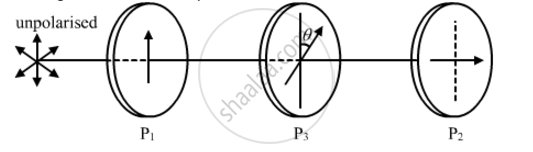# Two Polaroids P1 and P2 Are Placed with Their Pass Axes Perpendicular to Each Other. an Unpolarised Light of Intensity I0 is Incident on P1. - Physics

Two polaroids P1 and P2 are placed with their pass axes perpendicular to each other. An unpolarised light of intensity I0 is incident on P1. A third polaroid P3 is kept in between P1 and P2 such that its pass axis makes an angle of 30° with that of P1. Determine the intensity of light transmitted through P1, P2 and P3

#### Solution

As given in the question, the polaroids P1 and P2 are placed with their pass axes perpendicular to each other. Also, polaroid P3 is placed at an angle of 30° with respect to P1.Now, we have:

Intensity of light after falling on PI'=I_0/2

Intensity of light after falling on P3, I" =

I'cos^2(theta)=I_0/2cos^2(30^@)=(3I_0)/8

Therefore, a light of intensity (3I_0)/8will pass through the P3, and the angle between P3 and P2 will be 60° because of the condition given in the question.

Intensity of light after falling on P2, I"' = I" cos^2(theta)=(3I_0)/8cos^2(60^@)=(3I_0)/32

Concept: Polarisation
Is there an error in this question or solution?
2013-2014 (March) All India Set 1

Share